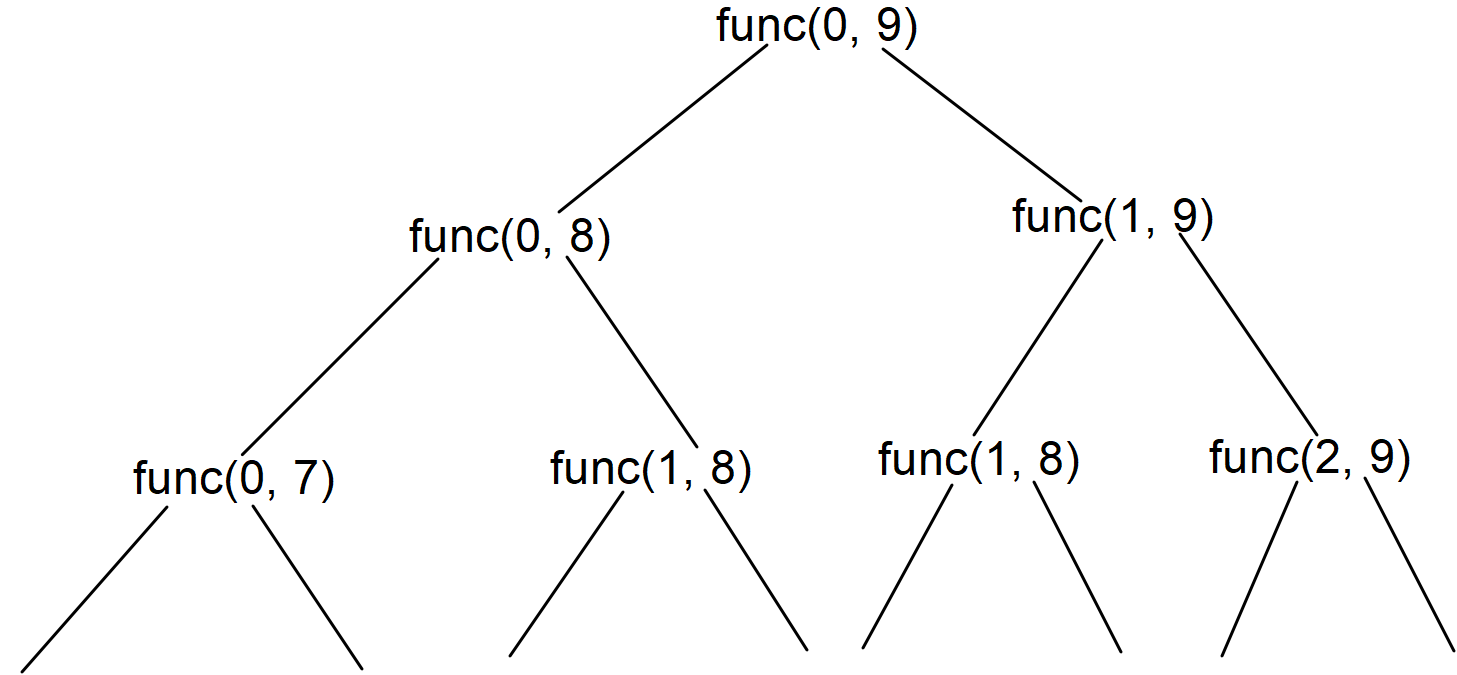# Longest Palindromic Subsequence

## Introduction:

We are given a String, and we need to find the length of the Longest Palindromic Subsequence.

Let us see few examples:

Input:

“ZXYFYKXAC”

Output: 5

Explanation:

“XYFYX” is the longest palindromic subsequence of length 5.

Input:

“ABBBBA”

Output: 6

Explanation:

“ABBBBA” is the longest palindromic subsequence of length 6.

## APPROACH 1:

We can use recursion to solve this question. We will keep two pointers, ‘i’ and ‘j’. The ‘i’ pointer will initially point at 0, and the ‘j’ pointer will initially point at ‘str.length()-1’. At any recursive call, if the character at ‘i’ and ‘j’ positions are the same, we will call our recursive function with the values ‘ i - 1’ and ‘j - 1’ and add 1 to the answer returned by this recursive call as we met a case for a string to be a palindrome.

In the else part, when the characters at the ‘i’ and ‘j’ positions are not the same, we will make two recursive calls with (i, j-1) and (i-1, j) because the elements at ith and jth position are not contributing into a palindromic sequence, and return the maximum among both of them.

Refer to the below implementation for the above approach.

Time Complexity: The time complexity for the above approach is 2ⁿ because in the worst case, we will either increment the ‘i’ pointer or decrement the ‘j’ pointer and make two choices.

Space Complexity:  The space complexity for the above approach is constant. We are not using any auxiliary space.

## APPROACH 2:

We can optimize APPROACH 1 by memoizing it. We can create a 2D D.P. table of size (str.length()) * (str.length()) to store the answer for each ‘i’ and ‘j’ value because it might be possible that we try to compute the answer for the same ‘i’ and ‘j’ values multiple times.

Refer to the below recursion tree.In the above recursion tree, we are calculating the value for func(1, 8) twice. Hence we can memoize it.

Refer to the below implementation for the above optimization.

Time Complexity: The time complexity for the above approach is O(N * N) because we are maintaining a DP table to store the answer for different (i, j) pairs. Hence, we will never calculate the answer for the already calculated (i, j) pair.

Space Complexity:  The space complexity for the above approach is O(N * N) because we maintain a 2D D.P. table of size O(N * N).

## APPROACH 3:

We can also write the above memoized recursive approach in an iterative manner using the bottom-up D.P. approach. We can create a 2D D.P. similar to the second approach of size (N * N) to store the answer for each ‘i’ and ‘j’ pair.

Time Complexity: The time complexity for the above approach is O(N * N) because we are running two nested for loops of O(N) time, making the overall time complexity to be O(N * N).

Space Complexity:  The space complexity for the above approach is O(N * N) because we maintain a 2D D.P. table of size O(N * N).

## FAQs:

What optimization did we do on the recursive approach for the Longest Palindromic Subsequence problem?

Since we were computing the answer for the same state at different times, we made a DP table to store the answer for the states we had already calculated. If we need the answer for the same state in the future, we can directly return the answer from the DP table.

What is the time complexity for the optimized approach of the Longest Palindromic Subsequence problem?

The time complexity for the optimized approach is O(N * N) because we are storing the answer for all possible ‘i’ and ‘j’ pairs, and if we have already calculated the answer for any recursion call, we return its answer stores in the DP table.

Can we apply a similar approach for the Longest Palindromic Subsequence problem if the array length has its maximum value of 10⁵?

If we apply a similar approach for the mentioned constraints, the compiler will give us the ‘Memory Limit Exceeded’ error because of forming the DP table.

## Key Takeaways:

In this blog, we have covered the following things:

1. We first discussed the Recursive approach to solve the Longest Palindromic Subsequence problem.
2. Then we saw how we optimized the approach by memoizing using the DP table.

Suppose you want to learn more about Dynamic Programming and practice some questions that require you to take your basic knowledge on Dynamic Programming a notch higher. In that case, you can visit our Guided Path for Dynamic Programming. To practice this problem, you can visit Practice Problem.BY: NISHANT RANA